# R S Aggarwal Solutions for Class 11 Maths Chapter 30 Statistics Exercise 30B

Class 11 Chapter 30 – Statistics exercise 30B solutions are provided here. All solutions have been prepared by our experts at BYJU’S which are accurate as per the latest syllabus. This exercise introduces students to a lot of questions related to variance and standard deviation of a discrete frequency distribution.

Class 11 Maths Chapter 30 Statistics Exercise 30B is based on the following topics:

• Variance
• Standard deviation
• Variance and standard deviation of a discrete frequency distribution

Variance and standard deviation Formulas: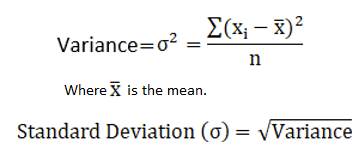## Download PDF of R S Aggarwal Solutions for Class 11 Maths Chapter 30 Statistics Exercise 30B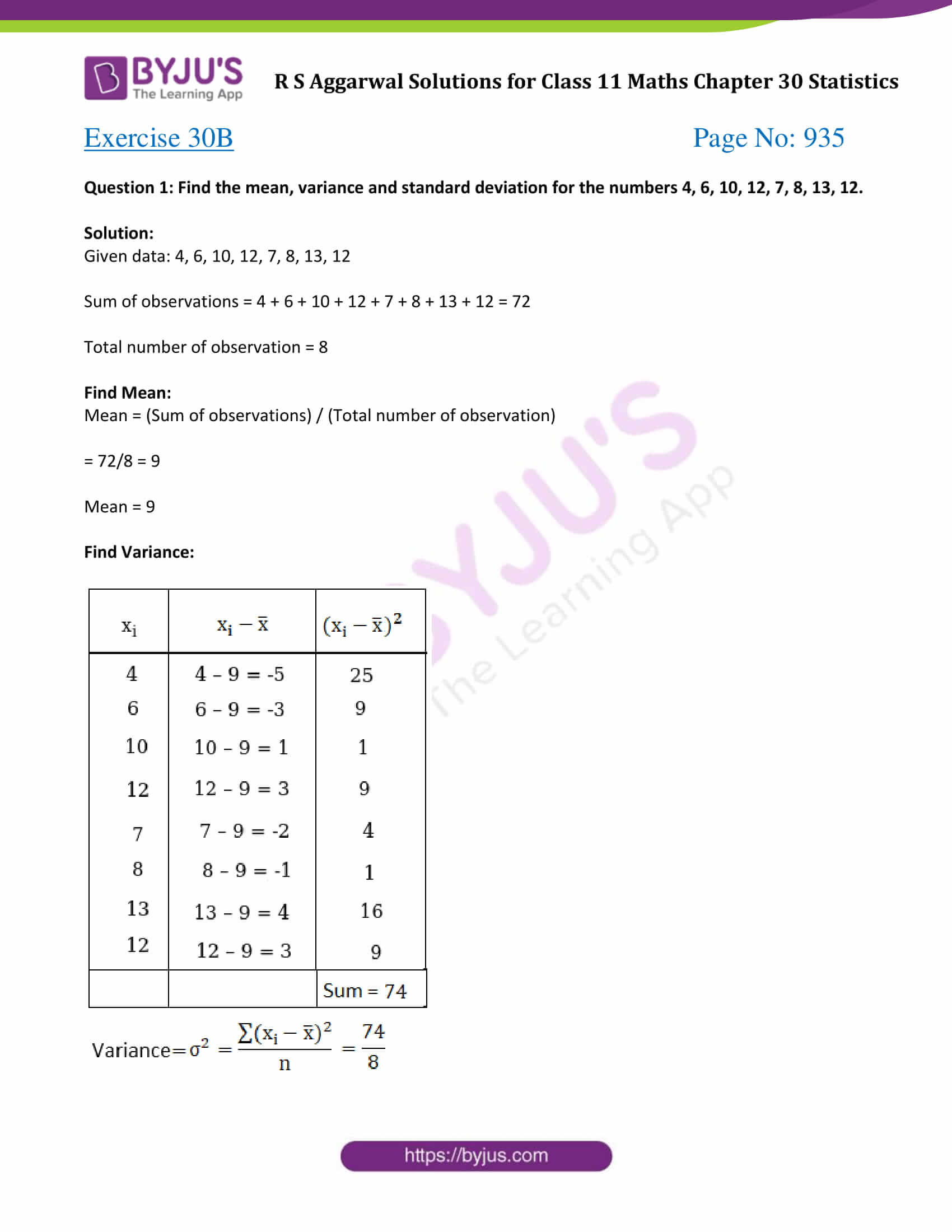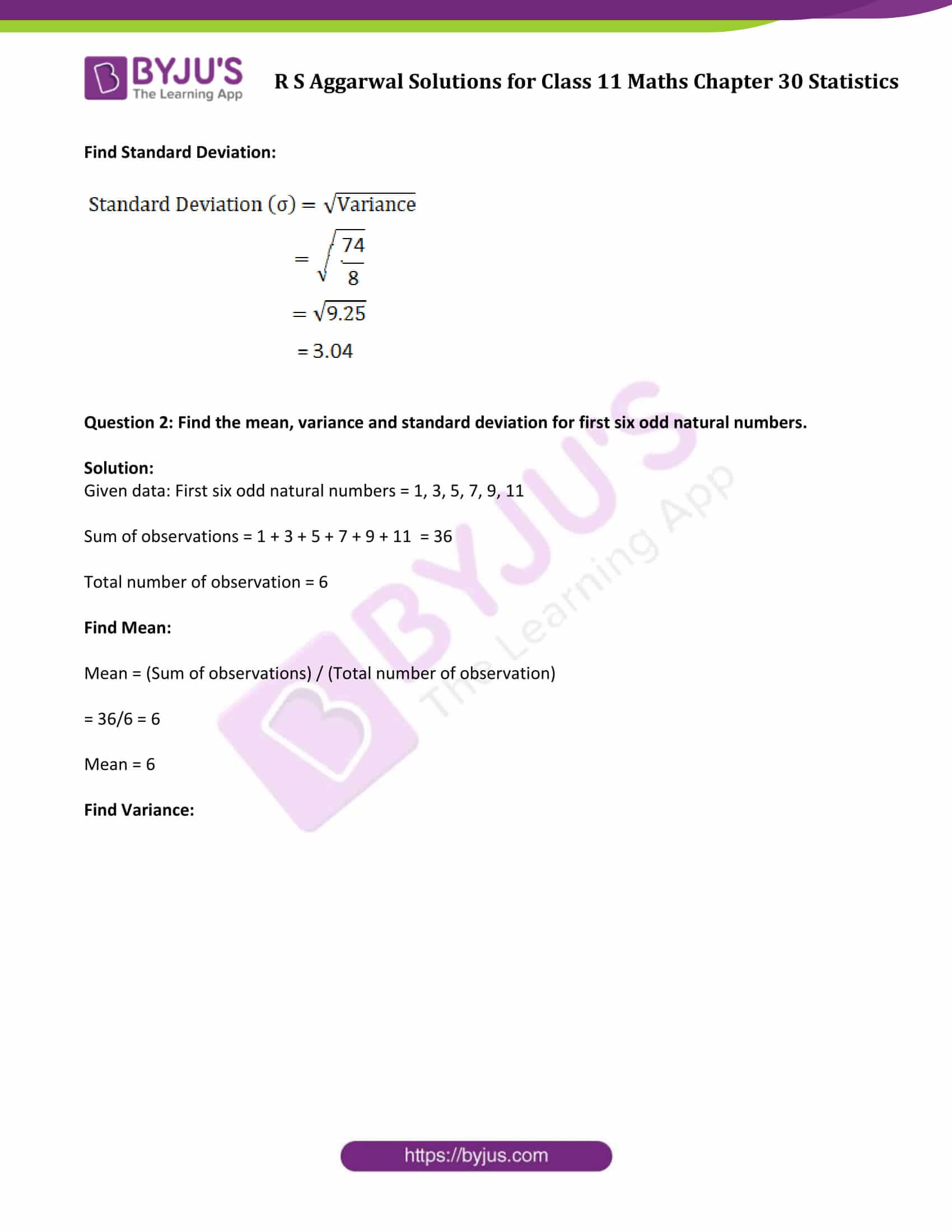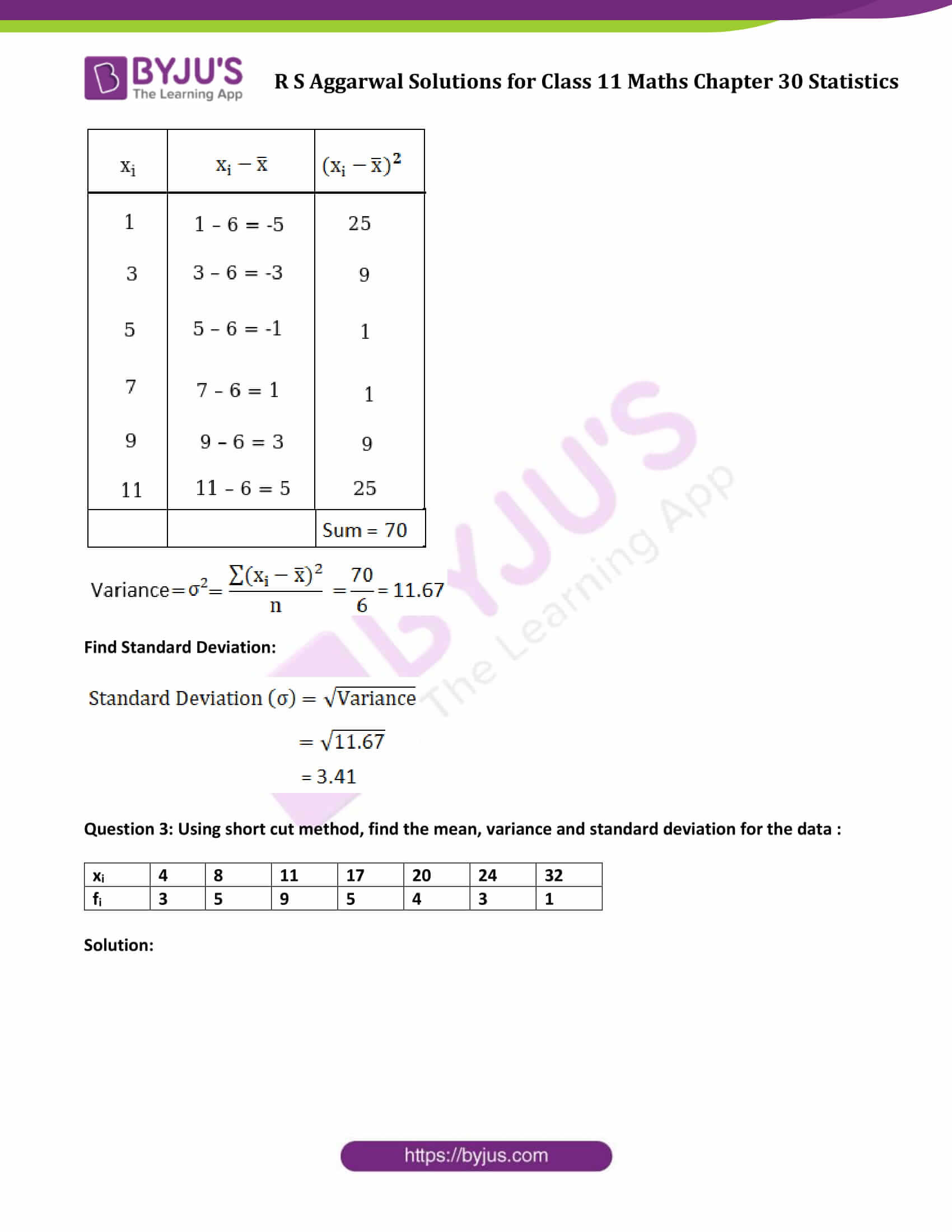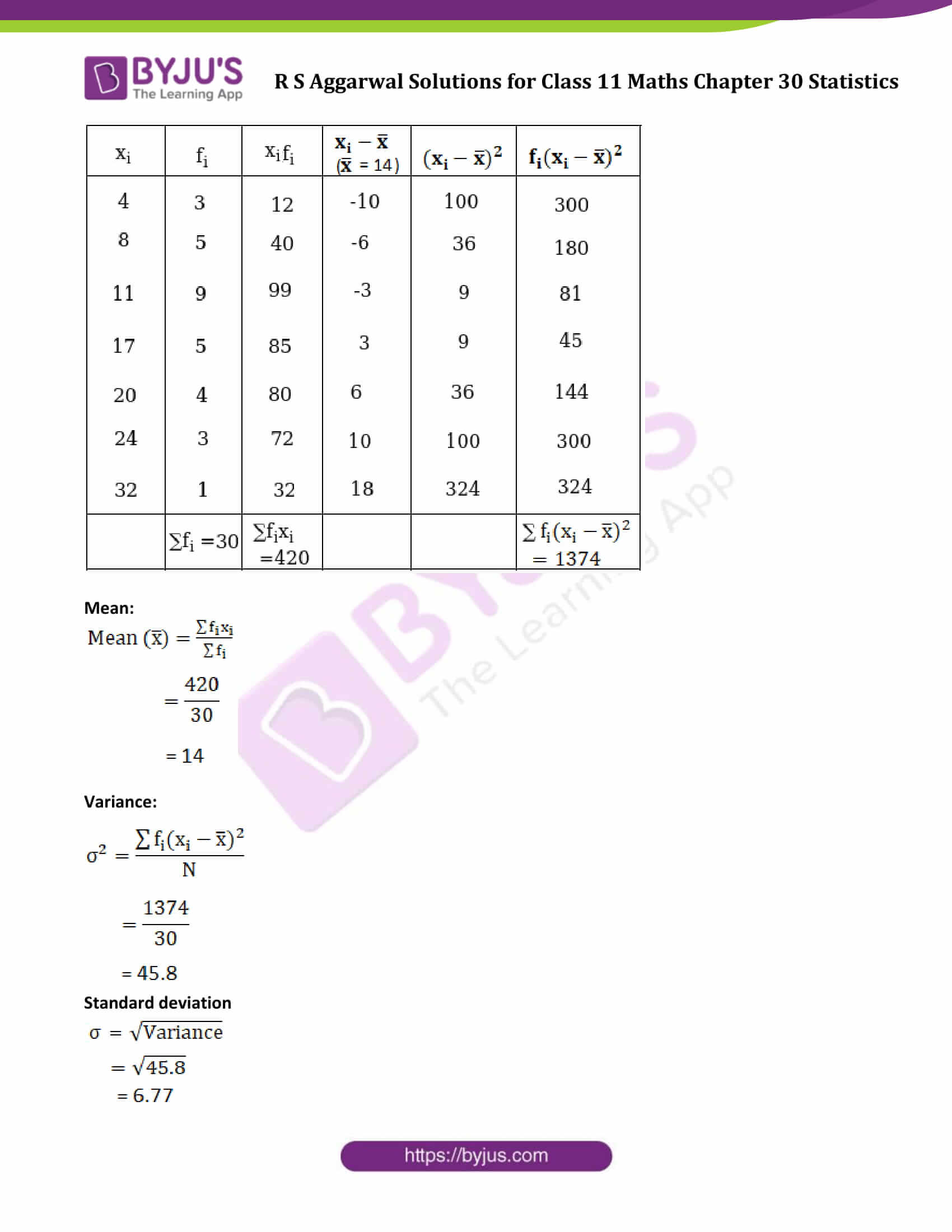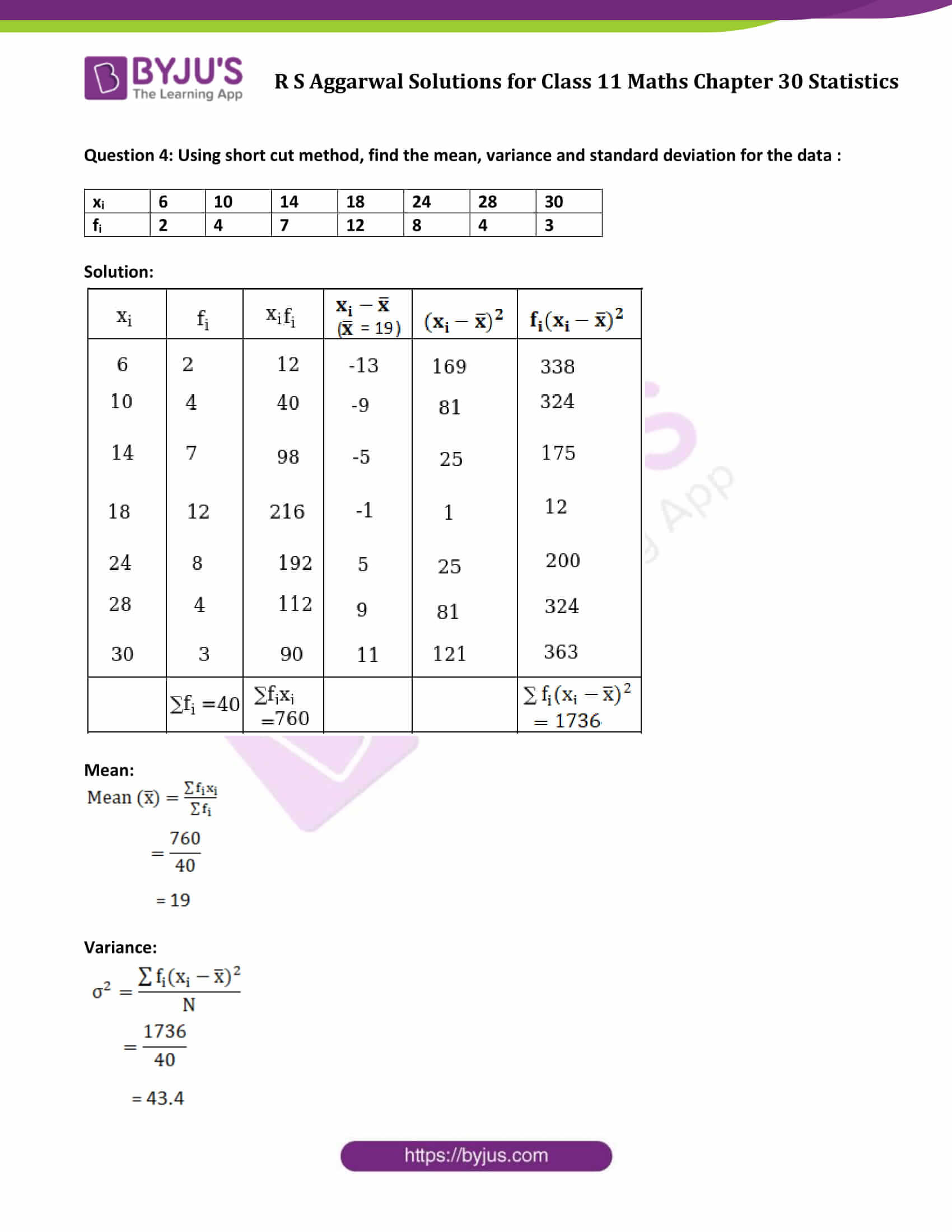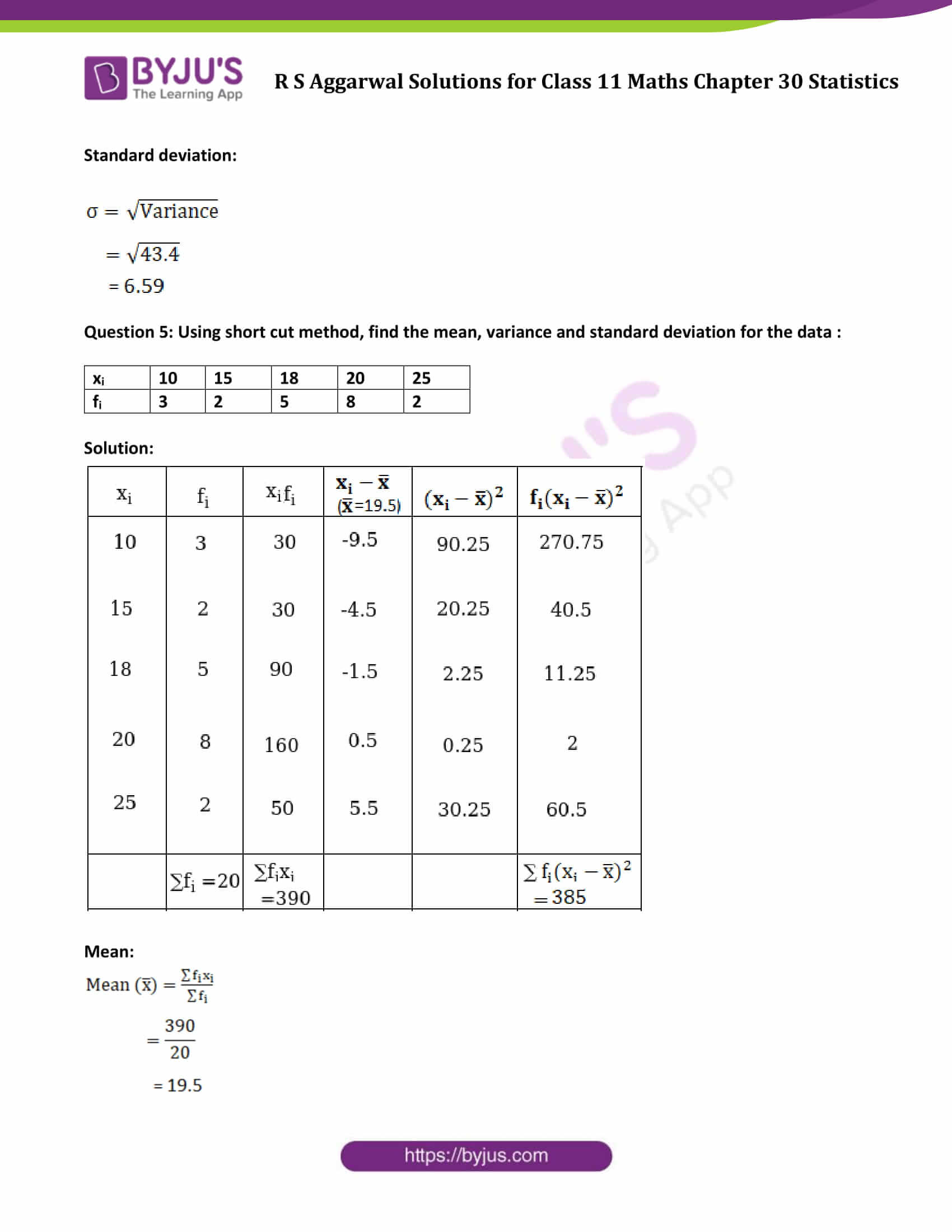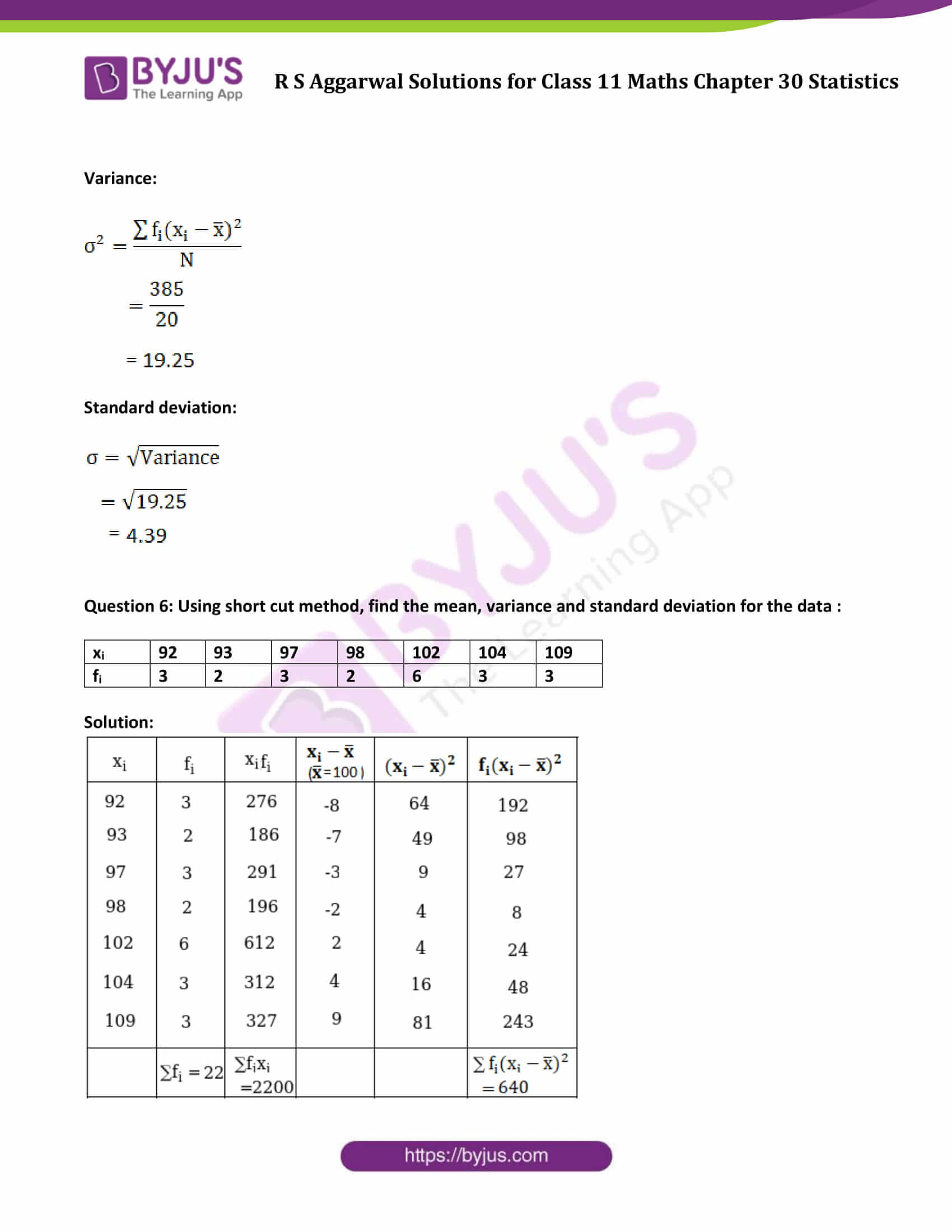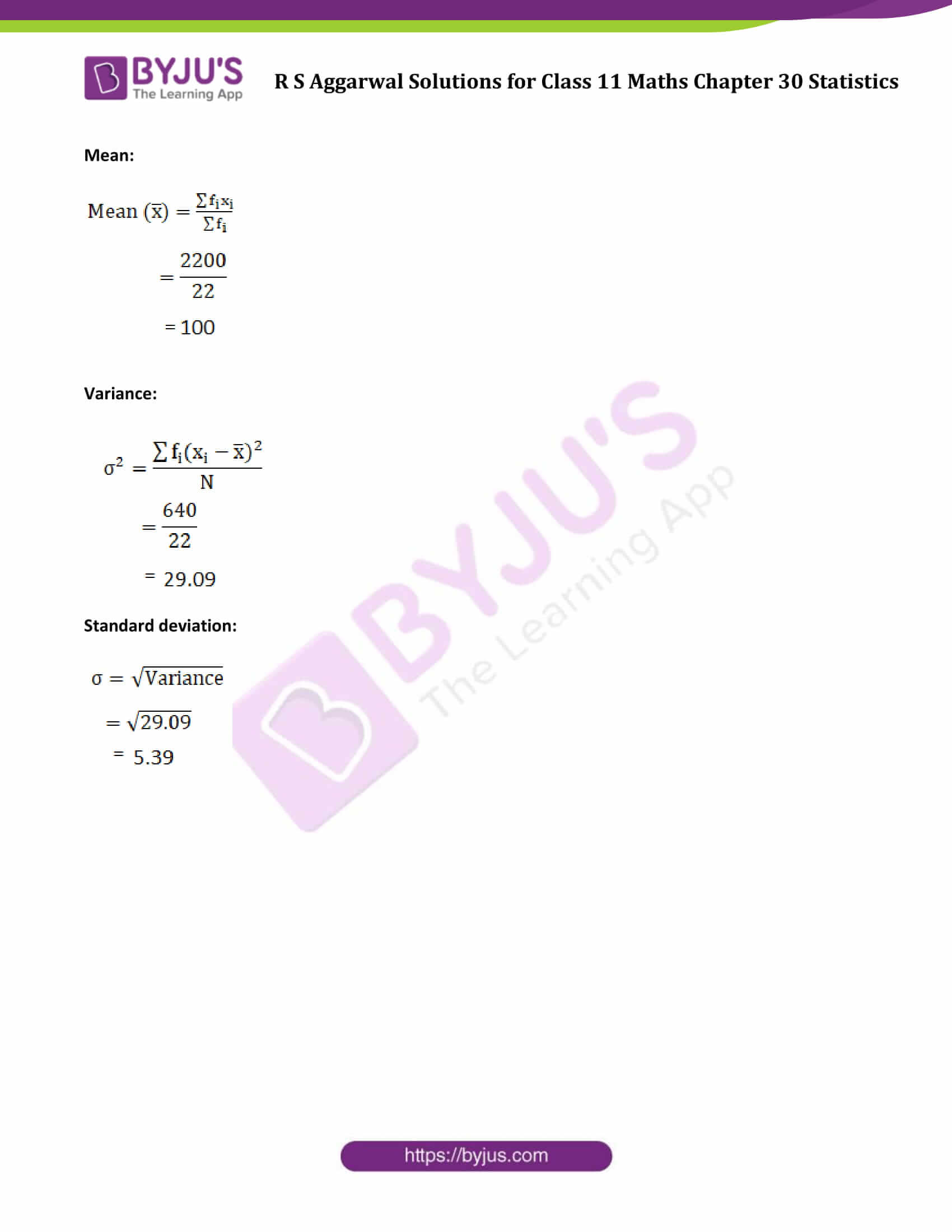### Access Answers to Maths R S Aggarwal Class 11 Chapter 30 Statistics Exercise 30B Page number 935

Question 1: Find the mean, variance and standard deviation for the numbers 4, 6, 10, 12, 7, 8, 13, 12.

Solution:

Given data: 4, 6, 10, 12, 7, 8, 13, 12

Sum of observations = 4 + 6 + 10 + 12 + 7 + 8 + 13 + 12 = 72

Total number of observation = 8

Find Mean:

Mean = (Sum of observations) / (Total number of observation)

= 72/8 = 9

Mean = 9

Find Variance: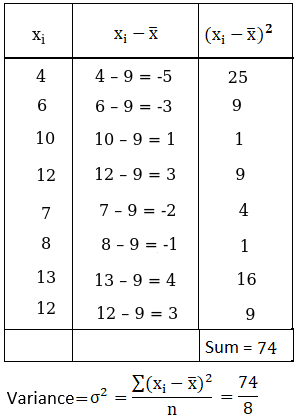Find Standard Deviation: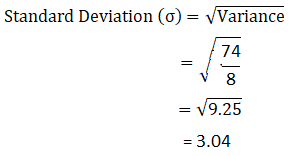Question 2: Find the mean, variance and standard deviation for first six odd natural numbers.

Solution:

Given data: First six odd natural numbers = 1, 3, 5, 7, 9, 11

Sum of observations = 1 + 3 + 5 + 7 + 9 + 11 = 36

Total number of observation = 6

Find Mean:

Mean = (Sum of observations) / (Total number of observation)

= 36/6 = 6

Mean = 6

Find Variance: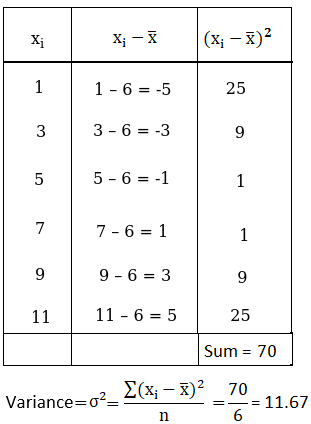Find Standard Deviation: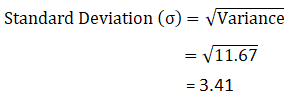Question 3: Using short cut method, find the mean, variance and standard deviation for the data :

 xi 4 8 11 17 20 24 32 fi 3 5 9 5 4 3 1

Solution: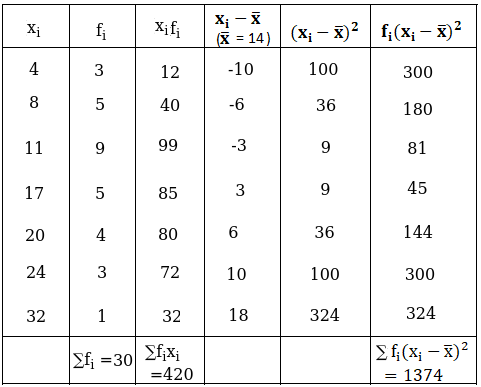Mean: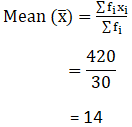Variance: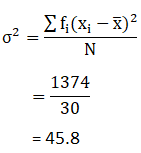Standard deviation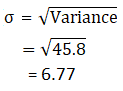Question 4: Using short cut method, find the mean, variance and standard deviation for the data :

 xi 6 10 14 18 24 28 30 fi 2 4 7 12 8 4 3

Solution: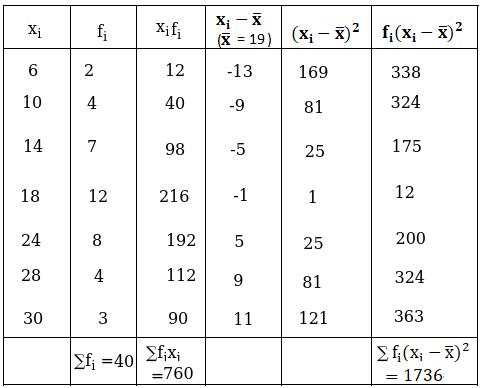Mean: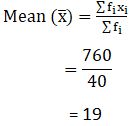Variance: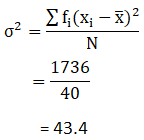Standard deviation: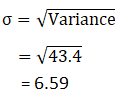Question 5: Using short cut method, find the mean, variance and standard deviation for the data :

 xi 10 15 18 20 25 fi 3 2 5 8 2

Solution: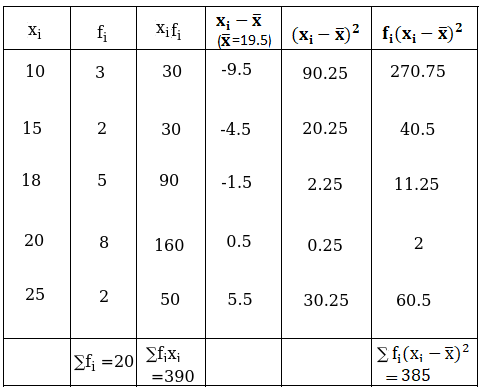Mean: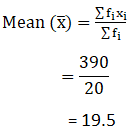Variance: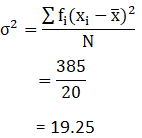Standard deviation: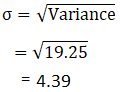Question 6: Using short cut method, find the mean, variance and standard deviation for the data :

 xi 92 93 97 98 102 104 109 fi 3 2 3 2 6 3 3

Solution: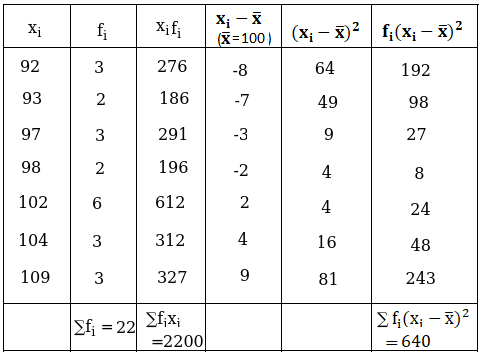Mean: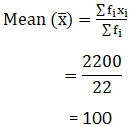Variance: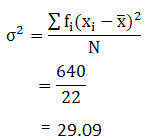Standard deviation: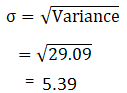## Access other exercise solutions of Class 11 Maths Chapter 30 Statistics

Exercise 30A Solutions

Exercise 30C Solutions

Exercise 30D Solutions